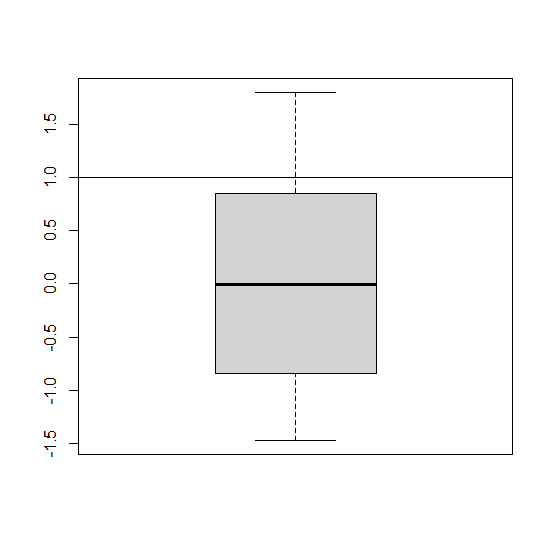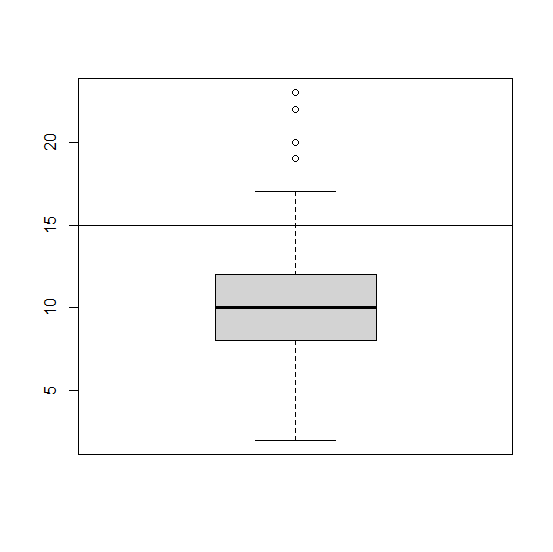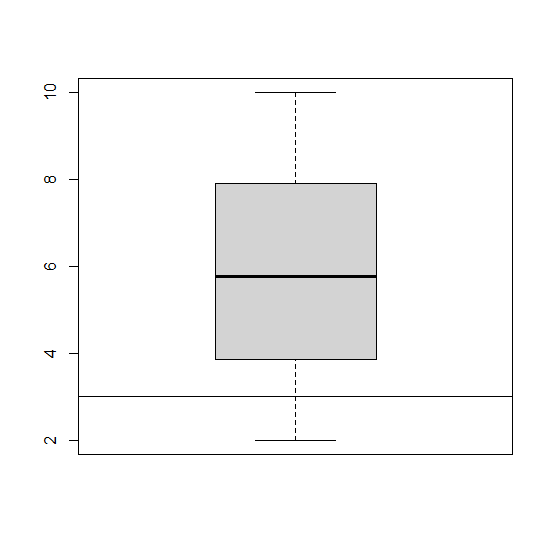# How to add a horizontal line in a boxplot created in base R?

A boxplot in base R already consists three horizontal lines that represents minimum, median, and the maximum but we might to create an extra horizontal to showcase some threshold value. For example, we might to create a horizontal line at 2 to understand the variation in values that are greater than say 2. This can be done very easily by using abline function after creating the boxplot.

## Example1

Live Demo

> x<-rnorm(10)
> boxplot(x)
> abline(h=1)

## Output:## Example2

Live Demo

> y<-rpois(500,10)
> boxplot(y)
> abline(h=15)

## Output:## Example3

Live Demo

> z<-runif(500,2,10)
> boxplot(z)
> abline(h=3)

## Output: